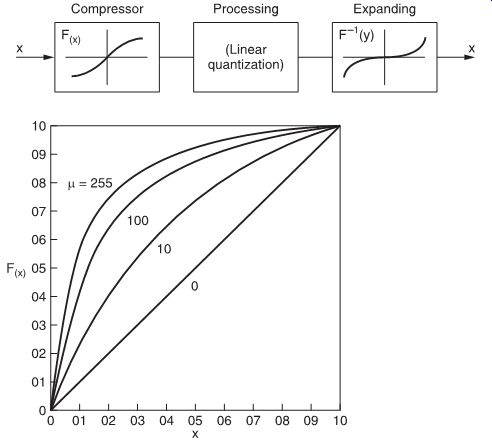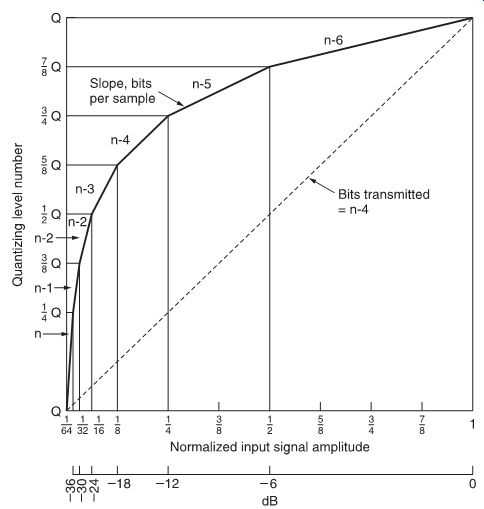# Digital Signal Processing: Overview of A/D conversion systems

 Home | Audio Magazine | Stereo Review magazine | Good Sound | Troubleshooting Departments | Features | ADs | Equipment | Music/Recordings | History
 Linear (or uniform) quantization In all the examples seen so far, the quantization intervals Q were identical. Such quantization systems are commonly termed linear or uniform. Regarding simplicity and quality, linear systems are certainly best. However, linear systems are rather costly in terms of required bandwidth and conversion accuracy. Indeed, a 16-bit audio channel with a sampling frequency of 44.056 kHz gives a bit stream of at least 16 × 44.056 = 705 × 10^3 bit s^-1 , which requires a bandwidth of 350 kHz--17.5 times the bandwidth of the original signal. In practice, a wider bandwidth than this is required because more bits are needed for synchronization, error correction and other purposes.FIG. 1 Non-linear quantization.FIG. 2 Characteristics of µ-law compressors. Since the beginning of PCM telephony, ways to reduce the band widths that digitized audio signals require have been developed. Most of these techniques can also be used for digital audio. Companding systems If, in a quantizer, the quantization intervals Q are not identical, we talk about non-linear quantization. It is, for instance, perfectly possible to change the quantization intervals according to the level of the input signal. In general, in such systems, small-level signals will be quantized with more closely spaced intervals, while larger signals can be quantized with bigger quantization intervals. This is possible because the larger signals more or less mask the unavoidably higher noise levels of the coarser quantization. Such a non-linear quantization system can be thought to consist of a linear system, to which a compander has been added. In such a system, the input signal is first compressed, following some non-linear law F(x), then linearly quantized, processed and then, after reconversion, expanded by the reverse non-linearity F-1 (y) (see FIG. 1). The overall effect is analogous to com panders used in the analog field (e.g., Dolby, dBx and others). The non-linear laws which compressors follow can be shown in graphical forms as curves. One compressor curve used extensively in digital telephony in North America for the digitization of speech is the 'µ-law' curve. This curve is characterized by the formula: Curves for this equation are shown in FIG. 2 for several values of µ. In Europe, the 'A-law' curve is more generally used (FIG. 3).FIG. 3 A-law characteristic curve. The (dual) formula for the 'A law' is: F(x) = Ax/1 + log A for 0 < x
Updated: Monday, 2019-08-26 15:01 PST